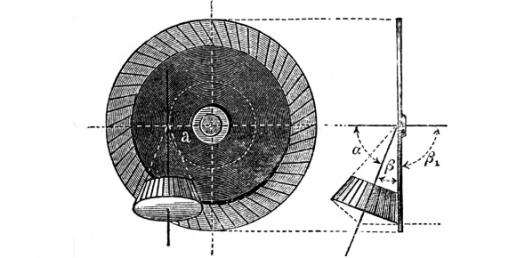# Kinematics Trivia Quiz: Speed,Velocity, And Acceleration!

5 Questions | Total Attempts: 419Settings.

• 1.
A car moves with a constant speed through 3 Km in 5 minutes. What is its speed?
• A.

10 m/s

• B.

0.6 m/s

• C.

1 m/s

• D.

600 m/s

• 2.
A car travels for 800 km in 20 hours. The details of the journey are shown below.DurationDistance Covered0 - 6 hours200 km6 - 8 hours0 km(at rest)8 - 16 hours400 km16 - 20 hours200 kmCalculate the average speed of the journey?
• A.

54.5 km/h

• B.

44.4 km/h

• C.

40 km/h

• D.

63.89 km/h

• 3.
An aeroplane moves with a constant speed of 150 m/s. Find the distance travelled in 2 minutes?
• A.

18 Km

• B.

1800 m

• C.

300 m

• D.

300 Km

• 4.
Lia runs at a constant speed of 4 m/s. What distance will he cover in 1 hr
• A.

14.4 Km

• B.

4 m

• C.

144 m

• D.

4 Km

• 5.
A car is at rest. What is its speed?
• A.

0 m/s

• B.

10 m/s

• C.

1 m/s

• D.

5 m/s

Related Topics